# Complete Guide To Graphs

## Graph Data Structure

Graph is a non-linear data structure defined as a collection of vertices(nodes) and edges(links).
These nodes are connected with the help of some edges. The nodes or vertices are used to store data.

A graph is represented as G = (V, E) where,

• V is the set of vertices or nodes
• E is the set of edges in the Graph G . It is represented as ordered pairs of vertices (i,j)

Example :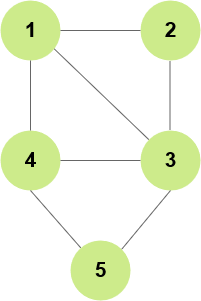Here:

``````V = {1, 2, 3, 4, 5}
E = {(1,2), (1,3), (1,4), (2,3), (3,4), (3,5), (4,5)}
``````

## Graph terminologies

• Distance between two Vertices : It is the number of edges present between any two vertices in the shortest path. If more than one edge is used to connect two vertices, then the shortest path is considered as the distance.

• Eccentricity of a Vertex : It is the maximum distance from a node or vertex to all other vertices.

• Adjacency: A vertex is said to be adjacent to another vertex if there is an edge connecting them.

## Types of Graph

### Directed and Undirected Graph

Undirected graph: A graph in which all the edges are bi-directional.
Edges do not point in any specific direction.
In an undirected graph, traversal can be done from a node_1 to node_2 or node_2 to node_1.

Total number of possible edges is `nC2` or `n(n-1)/2`

Directed graph: A graph in which all the edges are uni-directional that is, they point in one direction only. Traversal can only be done in one direction.

the total number of possible edges is `2(nC2)` or `n(n-1)`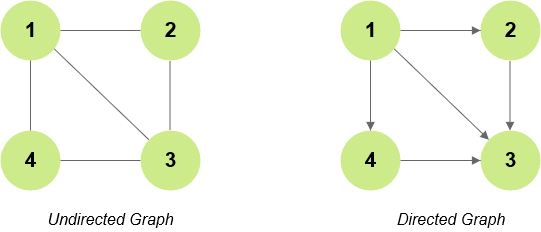### Weighted and Unweighted Graph

Weighted graph: There is some weight over the edges of the graph.

Unweighted graph: There is no weight over the edges of the graph.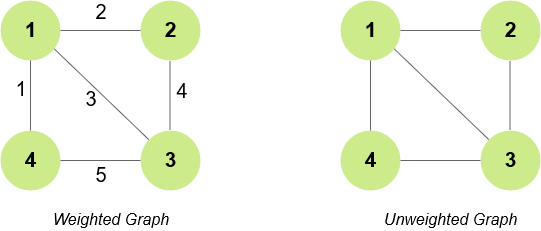### Connected and Disconnected Graph

Connected Graph In a connected graph from each node, there is a path to all the other nodes.
Disconnected Graph In a disconnected graph all the nodes can't be accessed from a particular node.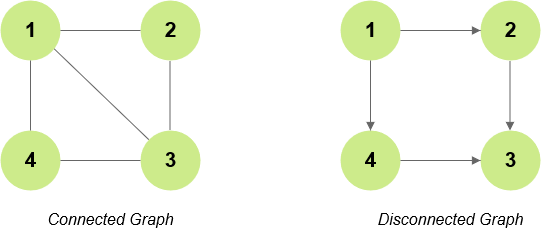## Graph Representation

There are two ways to represent the graph data structure:

• Adjacency matrix
• Adjacency list

### Adjacency Matrix

An adjacency matrix is a 2D array of V x V vertices. Each row and column represent a vertex.

`a[i][j]` = 1 – If there is an edge connecting vertex i and vertex j

`a[i][j]` = 0 – If there is no edge connecting vertex i and vertex j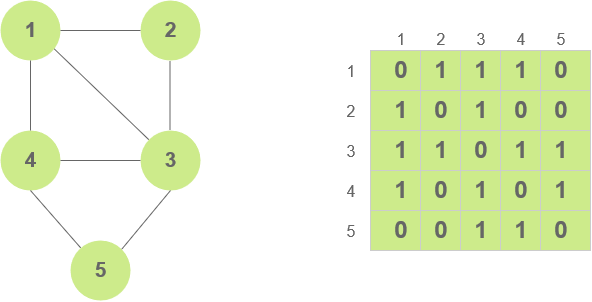Total space used : `O(V*V)`

### Adjacency List

Adjacency List is an array of linked list.
The size of the array is the number of vertices in the graph.
Indices of the array represent each node or vertex of the graph.
Each node or vertex has its own linked list and each node in this linked list contains the reference to the other nodes sharing an edge with the current vertex.
For weighted linked lists, the weights can be stored in the nodes of the linked lists.
Indices of the array represent each node or vertex of the graph.

If e is the number of nodes,

For an undirected graph, The total space used is `O(n + 2e)`

For an directed graph, the total space used is `O(n + e)`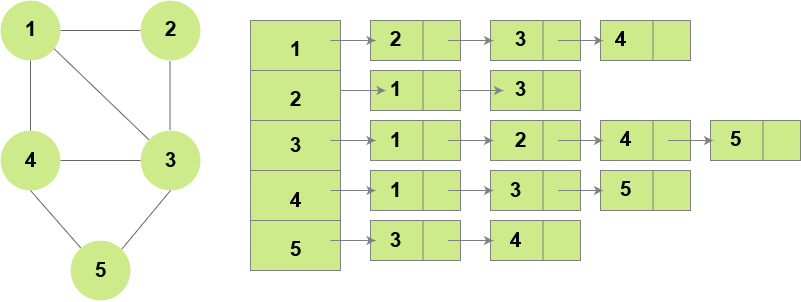Scroll to Top

### Full Stack Integrated Bootcamp Free Trial

• Please enter a number from 7000000000 to 9999999999.